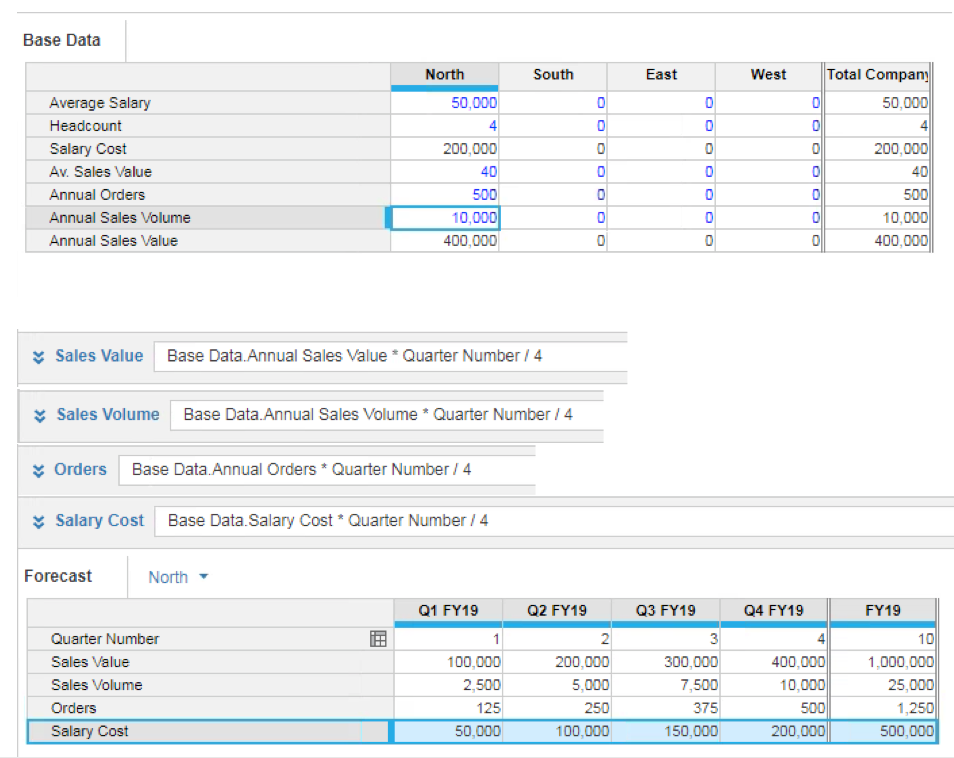# Getting data by line item into a dimension and generalising a complex formulaI want to calculate some values in my "Forecast" using a formula that is almost the same for each value. The only difference is that each row in the "Forecast" module gets an initial seed value from a different line item in the "Base Data" module.

The formula I want to use is actually a lot more complicated than shown in the example here, and instead of 4 rows, I have dozens, so I would like to not have to replicate the formula many times.

I've been working on doing this by building my "Forecast" module with a single Line Item, and moving the individual rows into a list. However, I can't work out how to write the formula in such a way that each list member in the "Forecast" module picks up the right value from "Base Data".

What I've got so far is:

1. Build a Line Item Subset from the Line Items in "Base Data"
2. Move the rows of the "Forecast" module to their own dimension
3. Add a List-type Line Item to the "Forecast" module so that I can associate each row in that module with its appropriate source row in "Base Data"
4. Create a single Line Item in the "Forecast" module whose job will be to to pull the appropriate value from the "Base Data" module and calculate the required results

But I can't see how to write the formula for the Line Item in step 3 so that it pulls the right value from "Base Data". If everything in "Base Data" was just data, it would be easy because I could set up "Base Data" with a single Line Item and move its rows into a list, but some of the rows in "Base Data" are formula-driven and therefore (I think) the rows in "Base Data" have to be Line Items.

Tagged:

•Hi Steve,

I would suggest that you stick with your original train of thought, and not use Line Item Subsets, because you mention the formula is ALMOST the same.

You certainly don't want to find yourself in a situation where you have to do 'If then else' for different members in the Line Item Subset, for example:

'If item(line item subset) = line item subset.sales value then A else if item(line item subset) = line item subset.sales volume then B else C'

Also note that you can only select 'Number' format line items to be part of your line item subsets, so there's some restriction in that sense.

However i don't get what you meant with "...but some of the rows in "Base Data" are formula-driven...", because Line Item subsets can still grab formula driven line items, so long as they are 'Number' format line items.

Thanks,

LipChean

•You are on the right lines, and you may have done some of this already, but I'll list the steps following best practice

1. Create a line item subset from your source module

2. Create a staging module using the above with a single line item (let's call it Value) with the COLLECT() formula; Turn the summaries off

3. Now you have a choice.

3a, If you want to map these to line items in the target then create a line item subset from the target module

3b. You could use a list in your target module if the "list" a simple aggregation

4. Create a mapping module using 1, and create a mapping line item formatted as either 3a or 3b

5. Enter the mapping for the source and targets

6. The target module then needs to be dimensioned by 3a or 3b with a single line item

7. The formula will be something like:

Staging module.Value[SUM:Mapping module,mapping]

As @LipChean_Soh  This can only be used for purely numeric items, but it is much more effective than multiple IFs, or manually re-pointing each formula

I hope this helps

David

•DavidSmithLipChean_Soh for the quick responses. @DavidSmith's solution got me results that I needed. I had to use LOOKUP instead of SUM in the final step, but I only mention this to help anyone else who comes across this in the future.

Thanks, both!

•Good stuff - Glad it worked for you